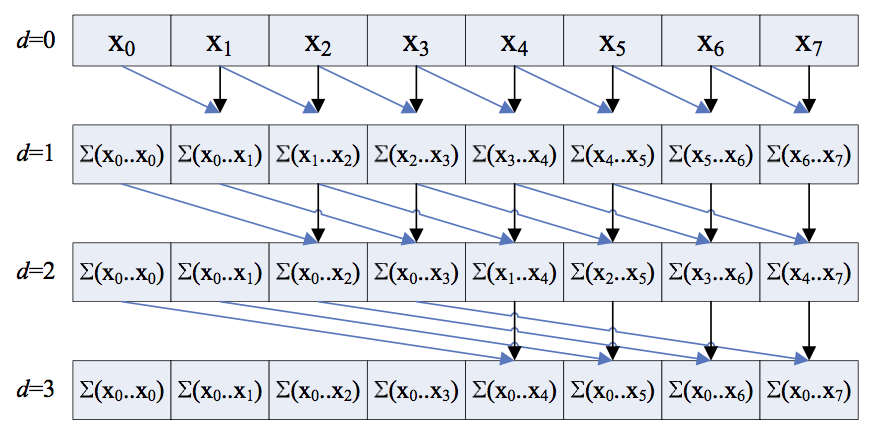Open in App
Not now

# Hillis Steele Scan (Parallel Prefix Scan Algorithm)

• Last Updated : 07 Jul, 2021

In this article, a scanning algorithm known as the Hillis-Steele Scan, also known as Parallel Prefix Scan Algorithm, is discussed. A scan operation in this context essentially means the calculation of prefix sums of an array. The Hillis-Steele scan is an algorithm for a scan operation that runs in a parallel fashion. Below is the approach of the algorithm for an array, x[] of size N:

• Iterate in the range [1, log2(N)] using the variable d and for all k in parallel, check if the value of k is at least 2d or not. If found to be true, then add the value of x[k – 2d – 1] to the value x[k].

Visual Representation:When depth d reaches log2N the calculation terminates and the result is calculated as the prefix sum of the array. All the individual additional operations run in parallel and each layer (d = 1, d = 2, …, ) proceeds linearly.

Below is the implementation of the algorithm in CUDA C++:

## C++

 `// C++ program for the above approach` `#include "cuda_runtime.h"``#include "device_launch_parameters.h"``#include ``#include ``#include ``#include ``using` `namespace` `std::chrono;``using` `namespace` `std;` `// Function to handle error``static` `void` `HandleError(cudaError_t err,``                        ``const` `char``* file,``                        ``int` `line)``{``    ``// If the error occurs then print``    ``// that error``    ``if` `(err != cudaSuccess) {``        ``printf``(``"\n%s in %s at line %d\n"``,``               ``cudaGetErrorString(err),``               ``file, line);` `        ``// Exit``        ``exit``(EXIT_FAILURE);``    ``}``}` `#define HANDLE_ERROR(err) (``HandleError(err, __FILE__, __LINE__))` `template` `<``typename` `T>``__global__ ``void``Hillis_Steele_Scan_Kernel(T* arr,`` ``__int64` `space,``                          ``__int64` `step,`` ``__int64` `steps)``{``    ``__int64` `x = threadIdx.x``                ``+ blockDim.x * blockIdx.x;` `    ``__int64` `y = threadIdx.y``                ``+ blockDim.y * blockIdx.y;` `    ``// 2D Kernel Launch parameters``    ``__int64` `tid = x + (y * gridDim.x``                       ``* blockDim.x);` `    ``// Kernel runs in the parallel``    ``// TID is the unique thread ID``    ``if` `(tid >= space)``        ``arr[tid] += arr[tid - space];``}` `template` `<``typename` `T>``T* Hillis_Steele_Scan(T* input, ``__int64` `N)``{``    ``__int64``* out;``    ``HANDLE_ERROR(``        ``cudaMallocManaged(&out,``                          ``(``sizeof``(``__int64``) * N)));` `    ``// 2D Kernel Launch Parameters``    ``dim3 THREADS(1024, 1, 1);``    ``dim3 BLOCKS;``    ``if` `(N >= 65536)``        ``BLOCKS = dim3(64, N / 65536, 1);``    ``else` `if` `(N <= 1024)``        ``BLOCKS = dim3(1, 1, 1);``    ``else``        ``BLOCKS = dim3(N / 1024, 1, 1);` `    ``__int64` `space = 1;` `    ``// Begin with a stride of 2^0``    ``__int64` `steps = ``__int64``(log2(``float``(N)));` `    ``// Log2N depth dependency of scan``    ``HANDLE_ERROR(cudaMemcpy(``        ``out, input, ``sizeof``(``__int64``) * N,``        ``cudaMemcpyDeviceToDevice));` `    ``// Copy Input Array to Output Array``    ``for` `(``size_t` `step = 0;``         ``step < steps; step++) {``        ``Hillis_Steele_Scan_Kernel<< > >(``            ``out, space, step, steps);` `        ``// Calls the parallel operation``        ``space *= 2;` `        ``// A[i] += A[i - stride]``        ``// log N times where N``        ``// is array size``    ``}` `    ``cudaDeviceSynchronize();` `    ``return` `out;``}` `// Driver Code``int` `main()``{``    ``__int64``* inputArr;``    ``__int64` `arraysize = 10;` `    ``// Size of the input array``    ``__int64` `N = ``__int64``(1)``                ``<< (``__int64``(log2(``float``(arraysize))) + 1);` `    ``// N is the nearest power of 2``    ``// to the array size``    ``cout << ``"\n\nELEMS --> 2^"` `<< N``         ``<< ``" >= "` `<< arraysize;` `    ``// Allocate memory on the GPU``    ``HANDLE_ERROR(cudaMallocManaged(&inputArr,``                                   ``(``sizeof``(``__int64``) * N)));` `    ``HANDLE_ERROR(cudaDeviceSynchronize());` `    ``// INIT Test Data``    ``for` `(``__int64` `i = 0; i < N; i++) {``        ``inputArr[i] = 1;``    ``}` `    ``// An array with only 1s was chosen``    ``// as test data so the result is``    ``// 1, 2, 3, 4, ..., N``    ``high_resolution_clock::time_point tg1``        ``= high_resolution_clock::now();` `    ``__int64``* out = Hillis_Steele_Scan(``        ``inputArr, N);` `    ``// Function Call``    ``high_resolution_clock::time_point tg2``        ``= high_resolution_clock::now();` `    ``duration<``double``> time_span``        ``= duration_cast >(tg2 - tg1);` `    ``cout << ``"\nTime Taken : "``         ``<< time_span.count() * 1000``         ``<< ``" ms"``;` `    ``cout << endl;``    ``for` `(``__int64` `i = 0; i < arraysize; i++)``        ``std::cout << ``'\t'` `<< out[i];``    ``std::cout << std::endl;` `    ``cudaFree(out);` `    ``// Free allocated memory from GPU``    ``cudaFree(inputArr);` `    ``return` `0;``}`

Complexity Analysis: O(log N) time and O(N) processors

My Personal Notes arrow_drop_up